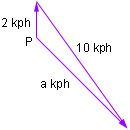Hi, my name is Sharon Ann and I am in my 2nd year at uni doing a degree in Maths. I am preparing for a resit and really need help with a few questions. Q: A motor boat is travelling in a southeasterly direction in water that is flowing from the south at 2km per hour. Show that the speed of the boat is (6 times the square root of 2) km per hour, given that it can travel at 10km per hour in still water. (NEWTONIAN MECHANICS COURSE) I would be really grateful for any help you could give me or the addresses of any other websites that could help. Thanks a million Sharon Hi Sharon. Draw a vector diagram to illustrate the velocities.The vector pointing north (from the south) is in the direction of the flow of the water. The vector labeled "a kph" is in the direction of the motion of the boat. The third vector points in the direction of the bearing of the boat. I have placed the vectors so that the vector sum of "the water vector" and the "boat bearing vector" is the "boat motion vector". The law of cosines can be used to find a, the speed of the boat. By the law of cosines 102 = 22 + a2 -2*2*a*cos(angle P) Since the ancle at P is 5 pi/4 (225o) its cosine is -1 over the root of 2. Substitute this value into the law of cosines expression and use the general quadratic to solve the resulting quadratic in a. I found two solutions, one was negative and the other was the solution you are looking for. Cheers, Penny Go to Math Central